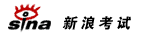# 2008年高考数学指导：解析几何专题热点复习

http://www.sina.com.cn 2008年03月21日 10:32   城市快报

天津市第四十二中学 张鼎言

2. 在平面直角坐标系xOy中，有一个以F1(0，--)和F2(0，-)为焦点、离心率为-的椭圆，设椭圆在第一象限的部分为曲线C，动点P在C上，C在点P处的切线与x、y轴的交点分别为A、B，且向量-=-+-。求：

(Ⅰ)点M的轨迹方程；

(Ⅱ)|-|的最小值。

解：(1)由已知，c=-，e=-=-，a=2，b2=1。

所以曲线C的方程为：

x2+-=1(x>0，y>0)

下面求过P点的曲线C的切线方程。

首先把曲线C的方程转化为函数形式，这是全题的关键。

∵y>0，∴y=2-，(0

直线方程斜率k=y'=--

因P(x0，y0)在曲线C上，有k=--，得切线AB的方程为：

y=--(x-x0)+y0

A(-，0)和B(0，-)，

由-=-+-，得M的坐标M(x，y)，x=-，y=-把P(x0，y0)代入曲线C的方程，得点M的轨迹方程为：

-+-=1(x>1，y>2)

(Ⅱ)|-|2=x2+y2=x2+-=x2+-+4=(x2-1)+-+59

当且仅当x2-1=-，x=->1时|-|的最小值为3。

3. 已知点A(x1，y1)，B(x2，y2)(x1x2≠0)是抛物线y2=2px(p>0)上的两个点，O是坐标原点，向量-，-满足|-+-|=|---|。设圆C的方程为x2+y2-(x1+x2)x-(y1+y2)y=0

(Ⅰ)证明线段AB是圆C的直径；

(Ⅱ)当圆C的圆心到直线x-2y=0的距离的最小值为-时，求P的值。

解：(Ⅰ)由|-+-|=|---|，∴(-+-)2=(---)2

整理得：-・■=0

∴x1・x2+y1・y2=0

AB中点为(-，-)，

-|AB|

=(-)2+-

=--

=-- (1)

圆C x2+y2-(x1+x2)x-(y1+y2)y=0

(x--)2+(y--)2

=(-)2+(-)2

=-[x12+x22+y12+y22+2(x1x2+y1y2)]

=-(x12+x22+y12+y22) (2)

对照(1)、(2)两式，问题得证。

(Ⅱ)先理解题意，圆C的圆心O'(-，-)是动圆，圆的半径长短也在变化，直线x-2y=0是定直线。问题的实质是动点到定直线的距离，问题转化为求动点的轨迹方程。

x=-=-

=-(y12+y22)

=-[(y1+y2)2-2y1y2]

=-[(2y)2-2y1y2]

又x1x2+y1y2=0

→-・■+y1y2=0

→y1y2=-4p2代入(*)式，整理得y2=p(x-2p)

更多高考信息请访问：新浪高考频道 高考论坛 高考博客圈

特别说明：由于各方面情况的不断调整与变化，新浪网所提供的所有考试信息仅供参考，敬请考生以权威部门公布的正式信息为准。[高　考]高考进行时(音频) 直击08艺考招生
 [其　他]中西有别：英汉文化八大差异

 网页 新闻

 ・《对话城市》直播中国 ・新浪特许频道免责公告 ・诚招合作伙伴 ・企业邮箱畅通无阻